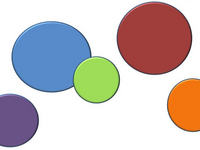Cody# Basics - Rounding

Curated by : AthiCommunity Group Solver

+50 points

Problem Title Likes Solvers Difficulty

#### Problem 42641. MATLAB Basic: rounding

Created by: Pritesh Shah

Tags function

17

1697

#### Problem 42642. MATLAB Basic: rounding II

Created by: Pritesh Shah

Tags function

9

1619

#### Problem 42643. MATLAB Basic: rounding III

Created by: Pritesh Shah

Tags function

11

1566

#### Problem 42644. MATLAB Basic: rounding IV

Created by: Pritesh Shah

Tags function

10

1582

#### Problem 2559. Check that number is whole number

Created by: Pritesh Shah

10

1463

#### Problem 2866. Matlab Basics - Rounding II

Created by: Yaz Majeed

9

1502

#### Problem 2867. Matlab Basics - Rounding III

Created by: Yaz Majeed

11

1377

#### Problem 2120. Rounding off numbers to n decimals

Created by: Marcel

Tags basic, easy, matlab

12

1447

#### Problem 43278. Make roundn function

Created by: Jang geun Choi

Tags easy, matlab, simple

16

1262

#### Problem 713. Find the maximum number of decimal places in a set of numbers

Created by: Vincent

27

951

#### Recent Activity

 3 hours and 3 minutes ago 3 hours and 8 minutes ago 3 hours and 15 minutes ago 17 hours and 43 minutes ago 17 hours and 43 minutes ago

### Community Treasure Hunt

Find the treasures in MATLAB Central and discover how the community can help you!

Start Hunting!